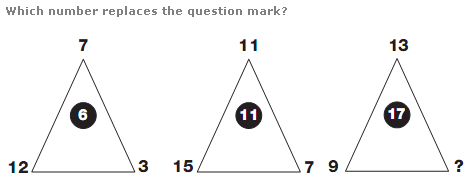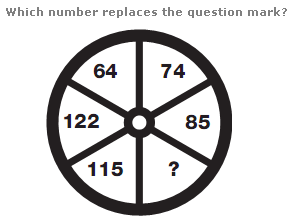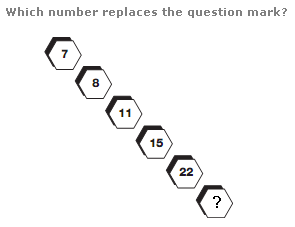# Puzzles - Number puzzles

### Exercise :: Number puzzlesAnswer : 13 Explanation : In each triangle, add up the lower 3 digits and divide by 3 to give the value at the apex of the triangle.Answer : 98 Explanation : Starting with the lowest number and moving clockwise, add the separate digits of the first number together and add this to the original number, writing the result in the next segment along.Answer : 33 Explanation : Add the first two numbers together and then subtract 4 to give the next number along.